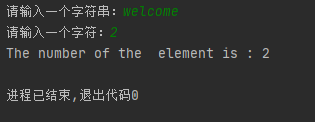Python实验之——列表和元组1387-常同学

热门标签

,

1. 编写程序，将前 6 个月份英文名存储在一个列表中，根据用户从键盘输入的月份数值，输出对应的月份英文名。
month=['January','February','March','April','May','June']
x = int(input('请 输 入 一 个 整 数： '))
if x>6 or x<0:
print("Error")
else:
print(month[x-1])

2.初始化一个列表（列表元素值可能重复），键盘输入一个数，判断这个数是否在列表中。如果在，输出每个出现的位置。否则，输出没找到。

import random
x=[random.randint(0,100) for i in range(20)]
print(x)
y=int(input('请 输 入 一 个 整 数： '))
if y not in x:
print("No Found")
else:
for i in range(0,20):
if x[i]==y:
print (i+1)

3.编写函数，接收包含 20 个整数的列表 lst 和一个整数 k 作为参数，返回新列表。处理规则为：将列表lst 中下标 k 之前的元素逆序，下标 k 之后的元素逆序，然后将整个列表 lst 中的所有元素逆序。

def news(lst,n):
if len(lst)!=20:
print(Error)
else:
if n<0 or n>18:
print(Error)
var1=lst[:n]
var2=lst[n:]
var1[::-1]
var2[::-1]
var3=var1+var2
var3= lst[::-1]
return var3

print(news([1,2,3,4,5,6,7,8,9,1,2,3,4,5,6,7,8,9,1,2],14))#测试函数

4.使用下面的函数头，编写一个函数找出字符串中某个特定字符的出现次数。要求不使用string 类提供的count 方法。编写程序实现提示用户输入一个字符串，然后再输入一个字符，调用该函数显示该字符在字符串中出现的次数，例如：count(“welocome”,2) 返回 2。
def count(s, ch):

def count(s,ch):
items=list(s)
ch=eval(ch)
a=items[ch-1]
num=0
for i in items:
if i== a:
num+=1
return num
def main():
s=input("请输入一个字符串：")
ch=input("请输入一个字符：")
print("The number of the  element is :",+count(s,ch))

main()5. 编写函数，接收一个包含若干整数的列表参数 lst，返回一个元组，其中第一个元素为列表 lst 中的最小值，其余元素为最小值在列表 lst 中的下标。

import random
def searchmin(lst):
m=min(lst)
tup=(m,)
for i,v in enumerate(lst):
if v==m:
tup=tup+(i,)
return tup

x=[random.randint(1,20) for i in range(10)]
print(x)
print(searchmin(x))

评论 抢沙发觉得文章有用就打赏一下文章作者

支付宝扫一扫打赏微信扫一扫打赏Vieu3.3主题Q Q 登 录